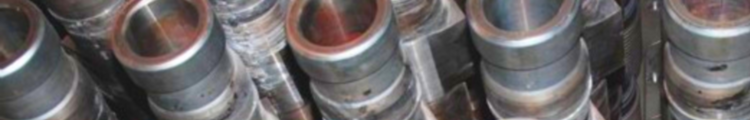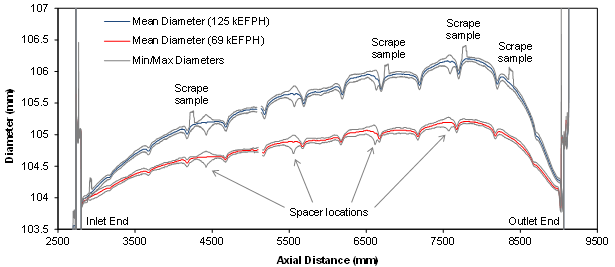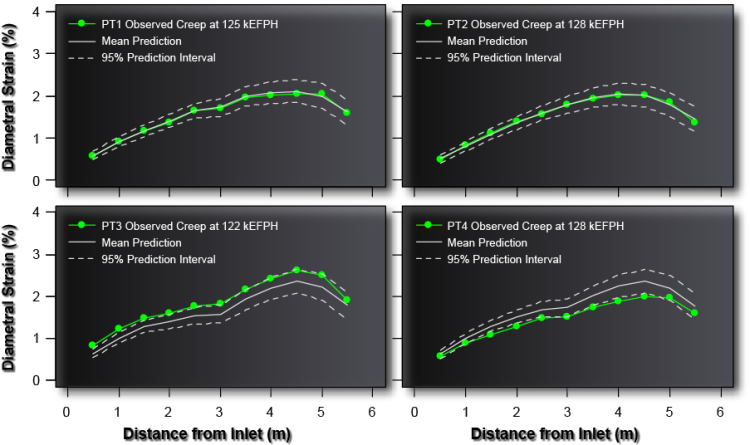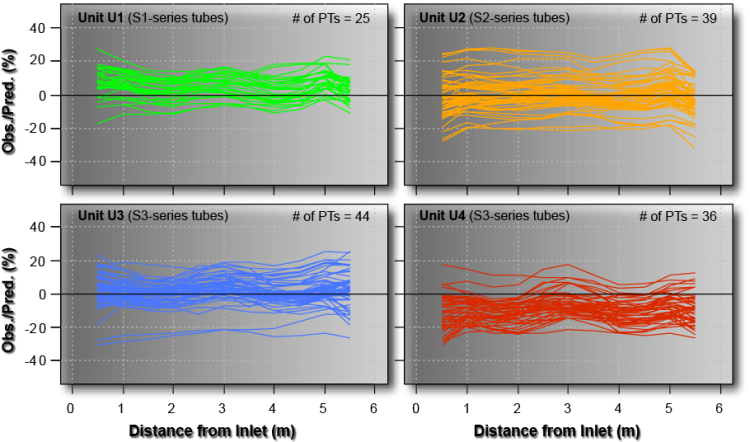## In-reactor diametral creep of Zr-2.5Nb pressure tubes

The cold-worked Zr-2.5 wt% Nb pressure tubes (PTs) constitute the pressure boundary within the core of CANDU reactors. Each pressure tube carries the primary heat transport fluid (heavy water) and encloses a string of fuel bundles. The pressure tubes are approximately 6 m long, 104 mm in diameter, and have a wall thickness of approximately 4.2 mm.

Understanding and predicting diametral creep in pressure tubes is vital for the lifetime management of CANDU reactors.  Diametral creep refers to the increase in PT circumference over time.  The key structural concern is stress rupture and creep ductility, while the main safety concern is related to the impact of flow bypass on fuel cooling.

The objective of this study was to develop a simplified statistical model for diametral creep using in-service inspection data from eight different CANDU reactor units.

### Data analysis

The data consists of operating conditions, such as temperature and flux, and corresponding CIGAR gauging records from a total of 328 in-service pressure tubes from various CANDU reactors.  Some tubes have been inspected multiple times, with inspection times ranging from 36,000 (effective full power hours) EFPH to 188,000 EFPH in some cases.### Model development

Diametral creep (%) in pressure tubes is modelled as linear in time and function of operating conditions as

\epsilon_i^{creep} = \beta_0 + \beta_1\phi_i t_i + \beta_2(T_i - T_0)^2 t_i + \beta_3\phi_i(T_i-T_0)^2t_i+\delta_i

where \phi is the fast neutron flux (×1017n m−2s−1, E ≥ 1 MeV), T is the temperature (K), T_0 is a constant reference temperature (K), t is the operating time (kEFPH), \beta_k are the regression coefficients, \delta_i is the error term associated with the i^{th} observation.

Because variance increases with diametral creep, the model was fitted using weighted least-squares (WLS), with the variance function as

\sigma_i^2 = \sigma_0^2(T_i-T_0)^2

where \sigma_0 is the standard error of regression.

### Model results• The predicted creep profiles are highly consistent with the observations along the axial length
• Similar predictions can readily be made for the uninspected pressure tube populations as a function of operating time

####• The tube specific residuals are highly consistent and nearly parallel with respect to axial distance
• This indicates that the WLS regression model is predicting the shape of the diametral creep profiles very well

### Summary and conclusions

• Pressure tube diametral creep increases as a linear function of operating time (this means that the lifetime of inspected tubes can be predicted with minimal uncertainty and very high confidence)
• The proposed WLS regression model can predict the shape of the diametral creep profiles very well
• The model is useful for predicting the end of life (EOL) of uninspected tubes and assessing tube-to-tube variability and manufacturing properties among the inspected tubes
• Future work includes constructing a "global" model to investigate the variability across different reactor units and other sub-populations, such as % recycled tubes, Task Group 3 tubes, etc.